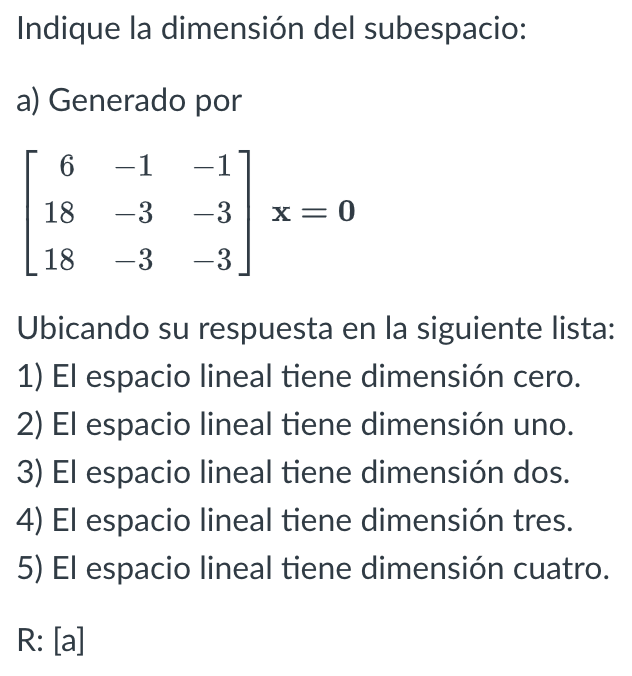# Question Indique la dimensión del subespacio: a) Generado por $\left[\begin{array}{rrr} 6 & -1 & -1 \\ 18 & -3 & -3 \\ 18 & -3 & -3 \end{array}\right] \mathbf{x}=\mathbf{0}$ Ubicando su respuesta en la siguiente lista: 1) El espacio lineal tiene dimensión cero. 2) El espacio lineal tiene dimensión uno. 3) El espacio lineal tiene dimensión dos. 4) El espacio Indique la dimensión del subespacio: a) Generado por $\left[\begin{array}{rrr} 6 & -1 & -1 \\ 18 & -3 & -3 \\ 18 & -3 & -3 \end{array}\right] \mathbf{x}=\mathbf{0}$ Ubicando su respuesta en la siguiente lista: 1) El espacio lineal tiene dimensión cero. 2) El espacio lineal tiene dimensión uno. 3) El espacio lineal tiene dimensión dos. 4) El espacio lineal tiene dimensión tres. 5) El espacio lineal tiene dimensión cuatro. $$\mathrm{R}:[a]$$Transcribed Image Text: Indique la dimensión del subespacio: a) Generado por $\left[\begin{array}{rrr} 6 & -1 & -1 \\ 18 & -3 & -3 \\ 18 & -3 & -3 \end{array}\right] \mathbf{x}=\mathbf{0}$ Ubicando su respuesta en la siguiente lista: 1) El espacio lineal tiene dimensión cero. 2) El espacio lineal tiene dimensión uno. 3) El espacio lineal tiene dimensión dos. 4) El espacio lineal tiene dimensión tres. 5) El espacio lineal tiene dimensión cuatro. $$\mathrm{R}:[a]$$
Transcribed Image Text: Indique la dimensión del subespacio: a) Generado por $\left[\begin{array}{rrr} 6 & -1 & -1 \\ 18 & -3 & -3 \\ 18 & -3 & -3 \end{array}\right] \mathbf{x}=\mathbf{0}$ Ubicando su respuesta en la siguiente lista: 1) El espacio lineal tiene dimensión cero. 2) El espacio lineal tiene dimensión uno. 3) El espacio lineal tiene dimensión dos. 4) El espacio lineal tiene dimensión tres. 5) El espacio lineal tiene dimensión cuatro. $$\mathrm{R}:[a]$$